# Undecidable Problems

## Mathematician Gareth Jones on Gödel’s incompleteness theorem, the halting problem and why the subsets of the natural numbers are uncountably infinite

### videos | April 23, 2020

I would like to tell you about undecidable problems. This is a topic in the theory of algorithms. In the early part of the 20th century mathematicians had a great sense of confidence that their subject could answer eventually any reasonable problem. This confidence was shattered in the 1930s by the announcement of several really surprising results. The first of these was Gödel’s incompleteness theorem. Kurt Gödel proved in 1931 that there are statements about the natural numbers, that is the positive integers, which are true but which can never be proved. It’s not that we don’t know a proof: he showed that we can never find a proof. And then a few years later Alonzo Church and Alan Turing independently showed that there are problems in mathematics which cannot be solved in the sense that there is no algorithm to solve them. Again, it’s not that we haven’t tried hard enough to find an algorithm: there is no algorithm to solve these problems.

I’ll give you a few examples in a few minutes but let me be a bit more precise about these problems. These are what are called decision problems.

A decision problem consists of a set of objects called instances (these could be numbers or equations, anything you like) together with a property that these objects may or may not have. The decision problem asks, does this particular object have the property we’re interested in?

For example, the objects could be integers, the property could be divisibility by 10, and we ask whether this integer is divisible by 10 or not. There is an easy algorithm to solve that problem: you just look at the last decimal digit, and if it’s 0, the integer is divisible by 10, if it’s not 0, it’s not divisible by 10. So that’s an algorithm which solves this problem. We say that a problem is decidable if there is an algorithm to solve it, an algorithm being a finite process which can be followed by any human being or any computer that is programmed to follow it and which always gives the correct answer: it must after a finite number of steps to produce the correct answer, yes or no.

What I would like to do first is give you an intuitive argument as to why one might expect some problems to be undecidable. The reason is that there are infinitely many problems one can ask, and there are infinitely many algorithms one can describe, but the infinite number of problems is bigger than the infinite number of algorithms. So there simply aren’t enough algorithms to solve all the problems. The algorithms form a set that is countably infinite. That’s mathematical jargon for saying that you can form these algorithms into a list, and there’s a first a second and a third member and so on: all algorithms will eventually appear in this list. You can do this with integers: for example, I can list all the integers. I can’t go ‘1, 2, 3’ and so on because then the negative numbers and 0 are left out. But I can go 0, 1, -1, 2, -2 and so on, and all the integers appear sooner or later in that list. In the same way, you can do this with the rational numbers. You cannot do it with the real numbers, there are too many of them to form them into a list.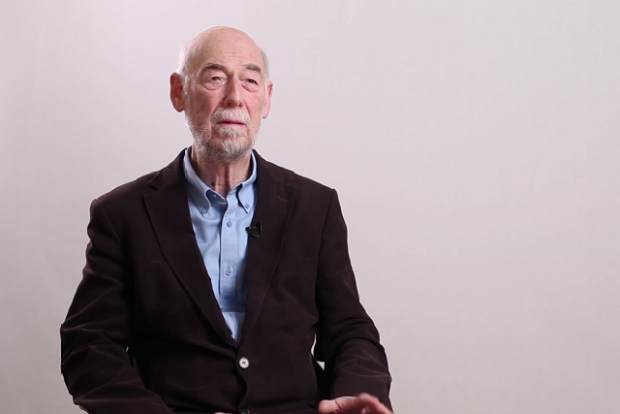Historian and Philosopher of Logic Stephen Read on the history of paradoxes, semantic paradoxes, and its direct connection to the foundations of mathematics
In a similar way, there are too many subsets of the natural numbers to form them into a list. We say that they are uncountably infinite, and I will try to prove this to you. It’s a slightly tricky argument, so please, pay attention. Let’s suppose that we could form all the sets of real numbers into a list, so there’s a first set, a second set, a third set and so on. For instance, we could have the even numbers as the first set, the odd numbers as the second set, the prime numbers as the third set and so on. Now let me define another set of natural numbers. I’ll call it S. It consists of all those numbers n which are not members of the n-th set. Again, S is the set of all integers n which are not in the n-th set Sn. Now, if we have listed all of the subsets, that set S that I have described must appear in the list, so it must be Sn for some n. And now we ask ourselves, is n a member of this set S? If it is, then because S equals Sn, n is a member of Sn. But that means it’s a member of S and therefore by definition of S it is not a member of Sn. And if n is not a member of Sn then it’s not a member of S, and by definition of S that means that n is not a member of Sn. So either way we’ve got a contradiction.

We’ve contradicted ourselves, and the contradiction must come from assuming that we could list all the subsets of the natural numbers. So we cannot do this listing process, there are too many subsets of natural numbers to form them into a list. They are uncountably infinite and that means that there are more of them then there are algorithms. We can write the algorithms in the list, we could just write them down in English and put them into dictionary order, just order them lexicographically: there’s no problem about that. But we cannot list all the subsets of the natural numbers and therefore we cannot list all the decision problems because for every subset of the natural numbers there is a decision problem: is this integer in that particular set? So there are at least as many decision problems as there are subsets of the natural numbers.

So I’ve tried to prove to you that there must be undecidable problems, but you will be unhappy about that because I haven’t given you an example: this does not produce a specific example of an undecidable problem. What Turing and Church did was produce concrete examples of such problems. I’ll very briefly describe Turing’s problem. He considered the halting problem.

An instance of the halting problem consists of a pair consisting of an algorithm and an input to that algorithm. The halting problem asks, if you run this algorithm with this input, will it eventually halt or will it run forever?

Let me give an example. Suppose, the algorithm starts with an integer, and the algorithm says ‘keep subtracting 1 until 0 appears and then halt’. Now, if I start that with a positive integer (say, 3) and run the algorithm, we’ll get 3, 2, 1, 0 and we’ll halt. If I start with a negative integer (say, -1) and run the algorithm, I keep subtracting 1 and I get -1, -2, -3 and it just goes on forever: we wait for 0 to appear and it never appears. So the algorithm does not halt with that particular input.

You could say we can solve this problem by just taking the algorithm and the input we are given and running the algorithm with that input and waiting to see what happens. If the algorithm halts we will see it halt and we will get ‘yes’ as an answer, but if the algorithm does not halt it will simply keep on running and we will be waiting for something to happen and we will never get a negative answer. So simply running the algorithm is not adequate, it doesn’t always give us an answer. Turing was able to prove by a rather ingenious method of contradiction that there can be no algorithm which solves this halting problem. There is no uniform way of describing, of determining whether a given algorithm with a given input will halt.

So the halting problem is unsolvable. I won’t describe Church’s undecidable problem: it’s algebra and it requires quite a lot of symbols to make it work. But what these two examples of undecidable problems do is they give rise to other undecidable problems in other areas of mathematics. Since the 1930s mathematicians have found examples of important problems in algebra, topology, in analysis and so on which are proved to be undecidable.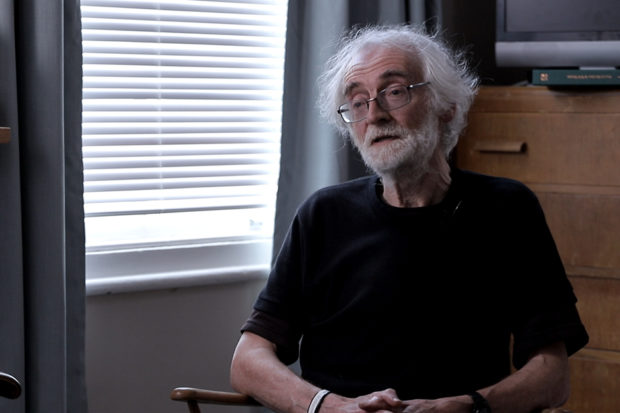Mathematician Gareth Jones on number theory, why there are infinitely many of prime numbers and how to calculate the proportion of prime numbers up to any given n
I’ll just finish with one specific example: this is Hilbert’s tenth problem. In 1900 to celebrate the new century Hilbert gave a talk in which he outlined a number of problems for 20th-century mathematicians to solve. Many of them have been solved. One of them, his tenth problem, was about Diophantine equations: these are polynomial equations with integer coefficients. Hilbert asked, if you are given a Diophantine equation, is there a solution in which the variables are all integers? And he wanted a uniform algorithm which would solve that problem.

Here’s an example. Let the equation be x2+y2=0. It’s easy to solve that: you can put x = y = 0, that gives you a solution in which the variables are all integers. Now, try x2+y2=3. You can solve it but not with integers: there are no integers x and y such as x2+y2=3. Just try it.

That particular polynomial is easy to deal with, but in 1971 a Russian mathematician Yuri Matiyasevich proved that there can be no algorithm which decides this question for all Diophantine equation. He did it building on earlier work by Davis, by Putnam and Robinson in the United States. It was a time when cooperation between Russia and the West was not very good but nevertheless, in subjects like mathematics, it’s occasionally possible for people in different nations to talk to each other. What Yuri Matiyasevich did was he used properties of the Fibonacci numbers to prove that there could be no algorithm to solve this problem. This is rather remarkable because the Fibonacci numbers are named after Fibonacci who was a 13th-century Italian mathematician Leonardo of Pisa. The Fibonacci numbers are normally named after him but in fact, they were discovered about five or six hundred years earlier by Indian mathematicians. It’s really rather remarkable at a very ancient mathematical structure, the Fibonacci sequence, was used to prove this very modern result that Hilbert’s tenth problem is undecidable.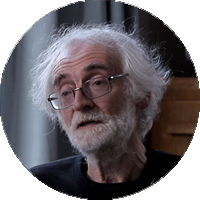Emeritus Professor of Mathematics, University of Southampton
Did you like it? Share it with your friends!
Published items
0779
To be published soon
+92

#### Most viewed

• 1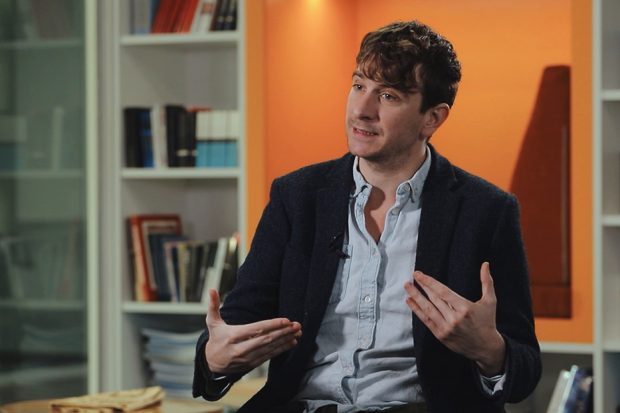Peter Jones
• 2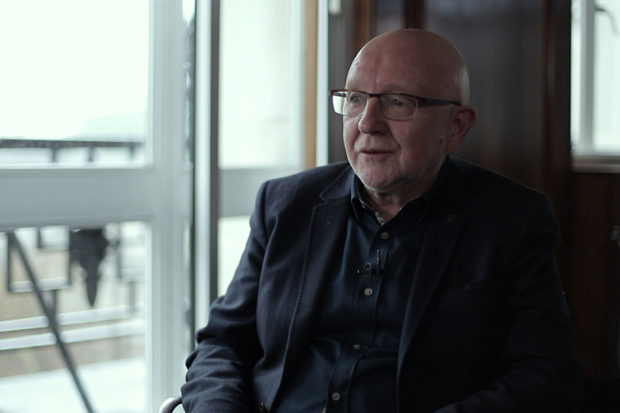John Worrall
• 3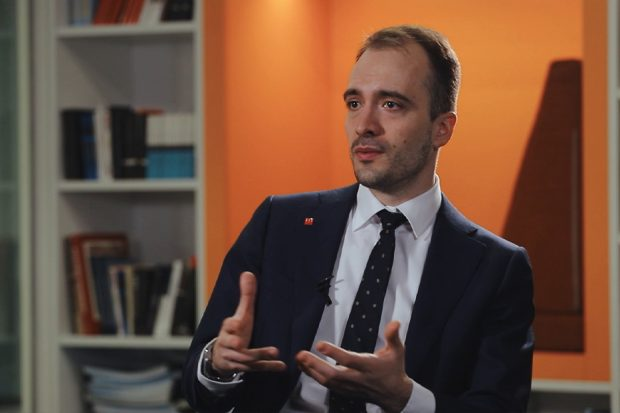Daniel Kontowski
• 4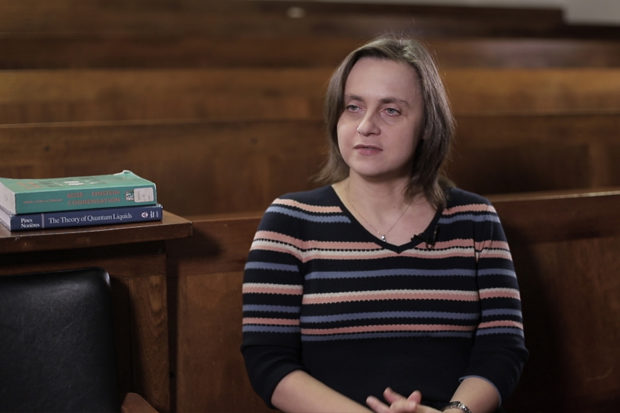Marzena Szymanska
• 5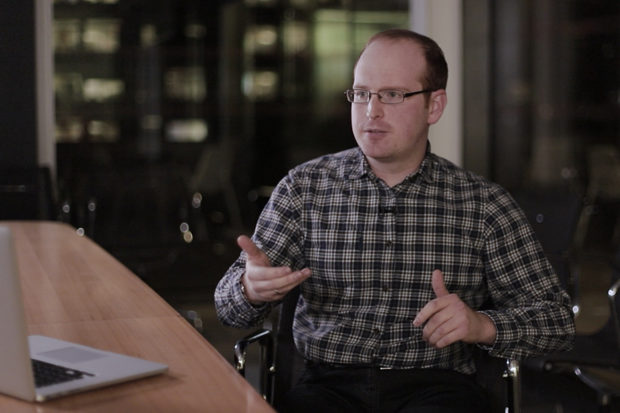Jonathan Birch
• 6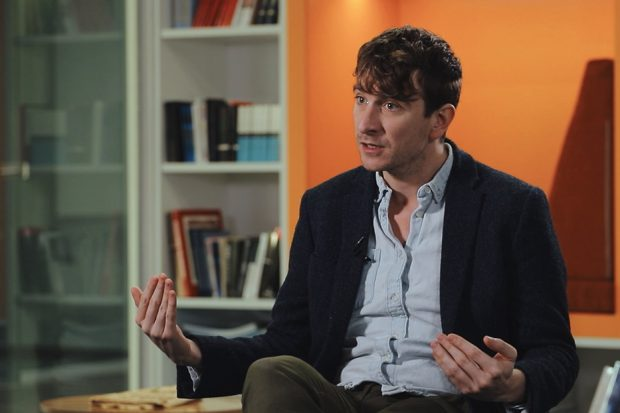Peter Jones
• 7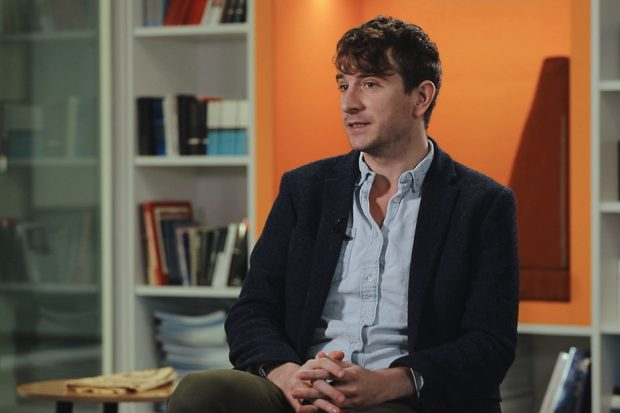Peter Jones
• 8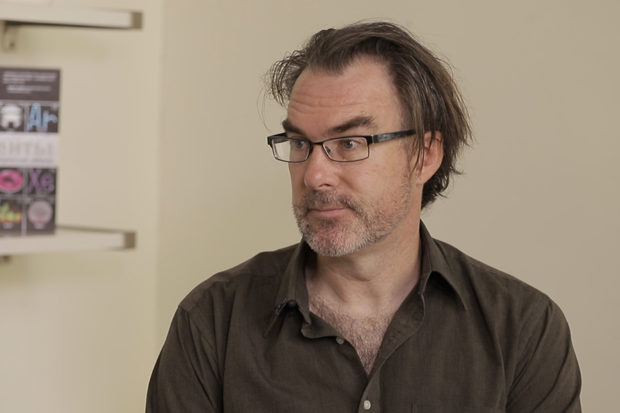Jeffrey Taylor
• 9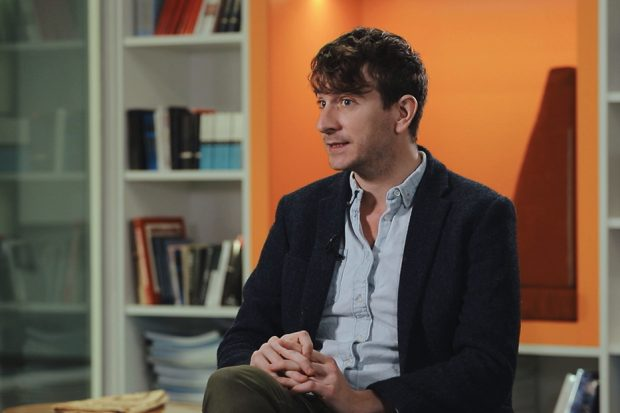Peter Jones
•